# Section A.2. GPS Receivers

### A.2. GPS Receivers

As in the previous section, we revisit the interference scenario considered in Figure 4-23 (here reproduced as Figure A-2) to describe the parameters used to derive equations for theoretical interference analysis of UWB systems on GPS receivers.

##### Figure A-2. Interference scenario of multiple UWB sources on a GPS receiver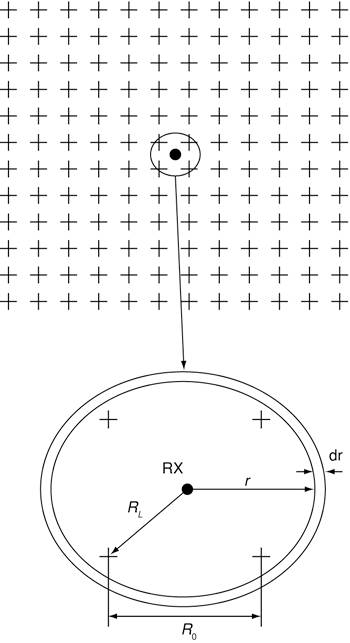The top half of the diagram in Figure A-2 shows multiple UWB transmitters, denoted by crosses, distributed about a 2D plane, and a cellular receiver at the position marked by a black dot. The bottom diagram is an enlarged version of the center of the top diagram, and it shows some of the dimensions required for the derivation of the interference power from the UWB transmitters to the receiver. The total interference power at the receiver from the UWB transmitters, IUWB, can be calculated by evaluating the following integral:

Equation A-9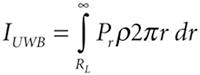where Pr is the received power from a UWB transmitter located at a distance r from the receiver. The distance from the receiver to the nearest UWB transmitters is RL, which can be expressed in terms of the average distance between UWB transmitters, R0, by

Equation A-10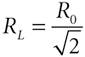The received power can be approximated by

Equation A-11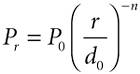where P0 is the power received at a free space reference distance d0 in the far-field region of a UWB transmitting antenna, and n is the path loss coefficient. It is appropriate to assume a d0 of 100 meters for urban macrocells. P0 can be expressed as

Equation A-12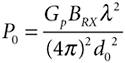Therefore, the total interference power at the receiver can be expressed as

Equation A-13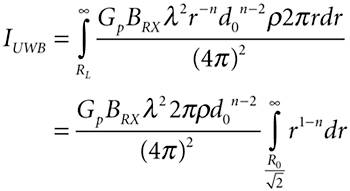Now provided 2 > n, the interference power is

Equation A-14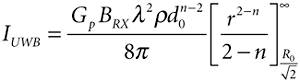Given that for 2 > n,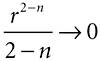as r, then the interference power at the receiver is

Equation A-15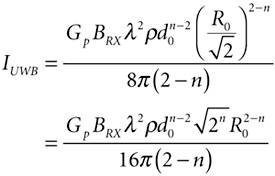The average distance between UWB transmitters is related to the density of UWB transmitters by

Equation A-16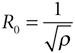Hence, the interference power, IUWB, can be expressed as a function of the density of UWB transmitters:

Equation A-17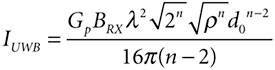For UWB transmitters distributed with density r such that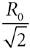is less than or equal to d0, then free-space propagation can be assumed from UWB transmitters within a distance of d0 from the cellular receiver. The preceding condition,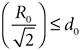can be rewritten as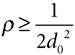When this condition is true, Equation A-9 becomes

Equation A-18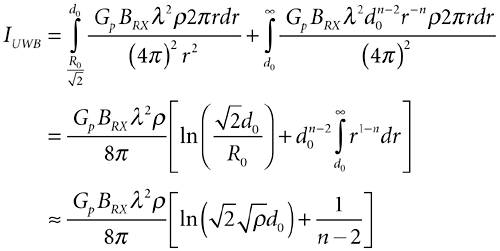A general expression for the UWB interference can be formulated by combining Equations A-17 and A-18:

Equation A-19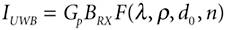where Gp denotes the ultra-wideband power spectral density, BRX represents the victim receiver's bandwidth, and F(l, r, d0, n)is described by

Equation A-20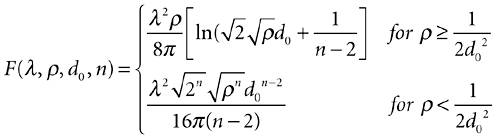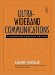Ultra-Wideband Communications: Fundamentals and Applications
ISBN: 0131463268
EAN: 2147483647
Year: 2005
Pages: 93

flylib.com © 2008-2017.
If you may any questions please contact us: flylib@qtcs.net